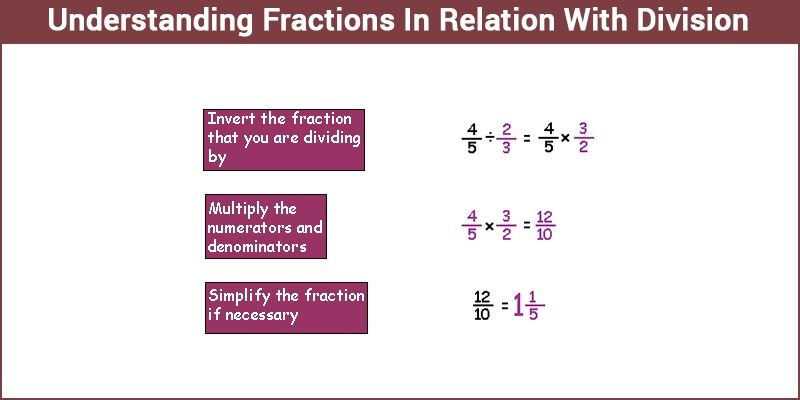# Understanding Fractions and Division

Fractions can turn into a factor of great fear and trepidation for children. But if one takes the time and effort to truly comprehend fractions there is no need to fear it. Fractions represent a part of the whole. A slash is generally used between two numbers to represent a fraction. The number on top is known as the numerator while the number below is named as the denominator. Take 1/5 for instance. The slash is what separates the numerator from the denominator. Here 1 ends up being numerator while 5 is relegated to the denominator.

A pizza is a wonderful example to explain fractions with. Take the pizza, all the slices of it are the denominator while the no of slices we end up getting is the numerator. So if you get 4 out of 8 slices, you represent that as a fraction like 4/8. Of course, there are proper and improper fractions along with like and unlike fractions.## Understanding Fractions In Relation With Division

When it comes to the division of fractions, there is a simple rule to remember that will help you solve all the problems related to the division of fractions. When you have two fractions to divide, just flip the second one and multiply and do not forget to simplify. But if the denominators between both the fractions that have to be divided are the same then just divide the first numerator by the second. One can see that if the second fraction is reversed and multiplied by the first fraction, it gets cancelled by each other and we are eventually left with the first numerator divided by the second.

## Video Lesson on Understanding Fractions